CAT  >  Practice Questions Level 3: Exponents & Logarithm

# Practice Questions Level 3: Exponents & Logarithm - Notes | Study Level-wise Practice Questions for CAT Preparation - CAT

 1 Crore+ students have signed up on EduRev. Have you?

This EduRev document offers 10 Multiple Choice Questions (MCQs) from the topic Exponents & Logarithm (Level - 3). These questions are of Level - 3 difficulty and will assist you in the preparation of CAT & other MBA exams. You can practice/attempt these CAT Multiple Choice Questions (MCQs) and check the explanations for a better understanding of the topic.

Question for Practice Questions Level 3: Exponents & Logarithm
Try yourself:What is the value of x + y in the following two equations?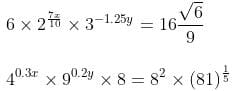Question for Practice Questions Level 3: Exponents & Logarithm
Try yourself:1 + loge z +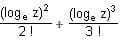+ ... is equal to

Question for Practice Questions Level 3: Exponents & Logarithm
Try yourself:The population of microbial bacteria "Gram staminee" increases exponentially as P(t) = aebt. The population of bacteria at the beginning of experiment at 10 a.m. was 20, which grows up to 40 by 12 p.m. What will be the population of bacteria at 3 p.m.?

Question for Practice Questions Level 3: Exponents & Logarithm
Try yourself:If 10x = x50, find the value of x.

Question for Practice Questions Level 3: Exponents & Logarithm
Try yourself:If P = log23 and Q = log69, which of the following options is true?

Question for Practice Questions Level 3: Exponents & Logarithm
Try yourself:If f(x) =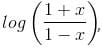then f(x) + f(y) is equal to

Question for Practice Questions Level 3: Exponents & Logarithm
Try yourself:If 46 + 12 + 18 + 24 + … + 6x = (0.0625)-84, then what is the value of x?

Question for Practice Questions Level 3: Exponents & Logarithm
Try yourself:The radioactive decay of a radioactive element is given as per the exponential function A(t) = A0 × eat, where t is half-life of that element and A0 is the amount of the element present initially. In a disastrous nuclear leakage incident in Chernobyl, in 1992, the level of plutonium-80 was found to be 20 times the safe level. If the half-life of plutonium-80 is 20 years, how long will it take for plutonium-80 level to reach the safe limits?

Question for Practice Questions Level 3: Exponents & Logarithm
Try yourself:Find the value of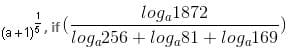loga961 = 1.

Question for Practice Questions Level 3: Exponents & Logarithm
Try yourself:The value of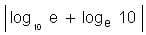is

The document Practice Questions Level 3: Exponents & Logarithm - Notes | Study Level-wise Practice Questions for CAT Preparation - CAT is a part of the CAT Course Level-wise Practice Questions for CAT Preparation.
All you need of CAT at this link: CAT

## Level-wise Practice Questions for CAT Preparation

277 docs
 Use Code STAYHOME200 and get INR 200 additional OFF

## Level-wise Practice Questions for CAT Preparation

277 docs

### How to Prepare for CAT

Read our guide to prepare for CAT which is created by Toppers & the best Teachers

Track your progress, build streaks, highlight & save important lessons and more!

,

,

,

,

,

,

,

,

,

,

,

,

,

,

,

,

,

,

,

,

,

;